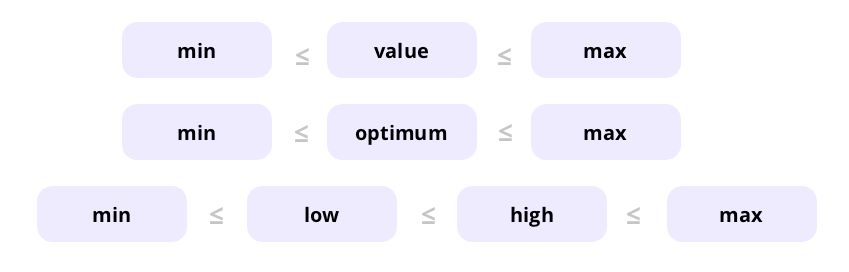Code has been added to clipboard!

# HTML meter Tag

Published Jun 29, 2017
Updated Nov 6, 2019

## HTML Meter: Main Tips

• HTML `meter` element is also known as a gauge.
• You can use it to represent a certain HTML scalar measurement in a predefined range.
• If you need to generate a progress bar, use <progress> tag instead.

## Using the Meter Tag

Which HTML element is used to display a scalar measurement within a range? The answer is simple - an HTML `meter`, or a gauge:

Example
``````
<p>Karma points: <meter optimum="30" high="80" max="100" value="85">85%</meter></p>
<p>Gas in Tanker: <meter low="20" max="100" value="11">11%</meter></p>
<p>Animals Petted: <meter low="10" high="60" min="0" max="50" value="43" title="Animals">Petting</meter></p>
<p>Satisfaction: <meter max="100" optimum="100" value="100">100%</meter></p>
``````

Note: the meter tag has been introduced in HTML5, so it's not supported by older versions.

## Tag-specific <meter> Attributes

HTML `meter` tag supports all global attributes and also has seven tag-specific ones:

• `value`, which you are required to set.
• `form`, which is used with meters associated with form controls.
• `high`, `low`, `max`, `min` and `optimum`, which define the meter range:We'll review each of the tag-specific attributes using code examples to further illustrate the point.

`value` defines the value of the meter to be displayed in numbers (0 by default):

Example
``<meter value="5" max="10"></meter>``

`form` indicates one or more form controls to which the HTML5 meter belongs:

Example
``````<label for="pass">Enter your exam score:</label>
<input type="button" value="Submit">

<p>The score you need to pass the exam:
<meter form="grade" min="0" low="20" high="80" max="100" value="70"></meter></p>``````

`max` sets a maximum number that is considered as the top of the range (1 by default):

Example
``````<meter max="100" value="95"></meter>
``````

`min` sets a minimum number that is considered as the bottom of the range (0 by default):

Example
``<meter min="0" value="100"></meter>``

`optimum` defines the optimum value for the meter:

Example
``<meter value="0.3" optimum="0.5"></meter>``

`high` sets a value that is to be considered as high. It must be lower than the value of `max` attribute and higher than the value of `min` and `low` attributes:

Example
``<meter min="0" max="100" high="80" value="50"></meter>``

`low` sets a value that is to be considered as low. It must be higher than the value of `min` attribute and lower than the value of `max` and `high` attributes:

Example
``<meter min="0" max="100" low="20" high="80" value="50"></meter>``

Note: high and low will only work if the values for min and max are set for the HTML5 meter.

## Browser support###### Chrome
6+###### Edge
All###### Firefox
16+###### IE
-###### Opera
11+6+

### Mobile browser support###### Chrome
18+###### Firefox
16+###### Opera
11+10.3+